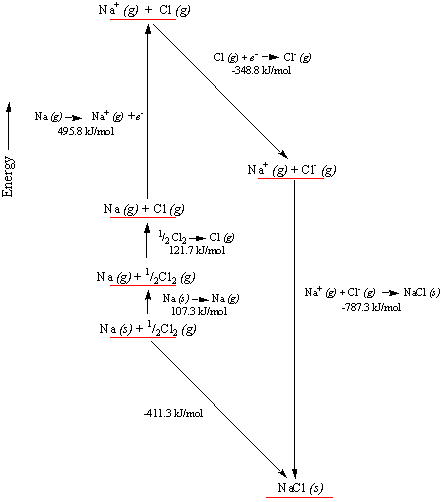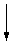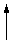Common Questions

Why Does?

Why Does Sodium Form NaCl?

Sodium reacts with chlorine to form Na+ ions and Cl- ions in spite of the fact that the first ionization energy of sodium is larger than the electron affinity of chlorine. To explain this, we need to divide the reaction between sodium and chlorine into a number of hypothetical steps for which we know the amount of energy given off or absorbed.

The starting materials for this reaction are solid sodium metal and chlorine molecules in the gas phase, and the product of the reaction is solid sodium chloride.

2 Na(s) + Cl2(g)2 NaCl(s)

Let's imagine that the reaction takes place by the following sequence of steps.

• A mole of sodium is converted from the solid to a gas. As might be expected, this reaction is endothermic.
 Na(s)Na(g)Ho = 107.3 kJ
• An electron is then removed from each sodium atom to form a mole of Na+ ions. The energy consumed in this reaction is equal to the first ionization energy of sodium.
 Na(g)Na+(g)Ho = 495.8 kJ
• A mole of chlorine atoms is formed by breaking the bonds in one-half a mole of chlorine molecules. Like the previous steps, this is an endothermic reaction.
 1/2 Cl2(g)Cl(g)Ho = 121.7 kJ
• An electron is then added to each chlorine atom to form a Cl- ion. This is the first exothermic step in this process, and the energy released is equal to the electron affinity of chlorine.
 Cl(g) + e-Cl-(g)Ho = -348.8 kJ
• The isolated Na+ and Cl- ions in the gas phase then come together to form solid NaCl. This is a strongly exothermic reaction, for whichHo is equal to the lattice energy of NaCl.
 Na+(g) + Cl-(g)NaCl(s)Ho = -787.3 kJ

If we consider just the first four steps in this reaction, Hess's law suggests that it 5.16 takes 376.0 kJ/mol to form Na+ and Cl- ions from sodium metal and chlorine gas.

 Na(s) + 1/2 Cl2(g)Na+(g) + Cl-(g)Ho = 376.0 kJ/mol

When we include the last step in the calculation, the lattice energy of NaCl is large enough to compensate for all of the steps in this reaction that consume energy, as shown in the figure below.

 Na(s) + 1/2 Cl2(g)NaCl(s)Ho = -411.3 kJ/molThe primary driving force behind this reaction is therefore the force of attraction between the Na+ and Cl- ions formed in the reaction, not the affinity of a chlorine atom for electrons.

Why does the reaction stop at NaCl? Why doesn't it keep going to form NaCl2 or NaCl3? The lattice energy would increase as the charge on the sodium atom increased from Na+ to Na2+ or Na3+. But to form an Na2+ ion, we have to remove a second electron from the sodium atom, and the second ionization energy of sodium (4562.4 kJ/mol) is almost 10 times as large as the first ionization energy. The increase in the lattice energy that would result from forming an Na2+ ion can't begin to compensate for the energy needed to break into the filled-shell configuration of the Na+ ion to remove a second electron. The reaction between sodium and chlorine therefore stops at NaCl.Why Does Magnesium Form MgCl2?

If the reaction between sodium and chlorine stops at NaCl, why does the reaction between magnesium and chlorine go on to form MgCl2? To answer this question, let's break the reaction into the following steps.

• A mole of magnesium is converted from the solid to a gas.
 Mg(s)Mg(g)Ho = 147.7 kJ
• An electron is removed from each magnesium atom to form a mole of Mg+ ions.
 Mg(g)Mg+(g)Ho = 737.7 kJ
• A second electron is then removed to form a mole of Mg2+ ions.
 Mg+(g)Mg2+(g)Ho = 1450.6 kJ
• Two moles of chlorine atoms are formed by breaking the bonds in a mole of chlorine molecules.
 Cl2(g)2 Cl(g)Ho = 243.4 kJ
• An electron is then added to each chlorine atom to form Cl- ions.
 2 Cl(g) + e-2 Cl-(g)Ho = -697.4 kJ
• The isolated Mg2+ and Cl- ions in the gas phase then come together to form solid MgCl2.
 Mg2+(g) + 2 Cl-(g)MgCl2(s)Ho = -2526 kJ

The lattice energy for MgCl2 is large enough to compensate for the energy it takes to remove the second electron from a magnesium atom because we don't have to break into a filled-shell configuration to form Mg2+ ions.

 Mg(s) + Cl2(g)MgCl2(s)Ho = -644 kJ/mol

The reaction stops at MgCl2, however, because it would take an enormous amount of energy to break into the filled-shell configuration of the Mg2+ ion to remove another electron.Why Do Semimetals Exist?

Metals have some or all of the following properties.

• They have a metallic shine or luster.
• They are typically solids at room temperature.
• They are malleable and ductile.
• They conduct heat and electricity.
• They exist as extended planes of atoms.
• They combine with other metals to form alloys, which behave like metals.
• They form positive ions, such as the Na+, Mg2+, Fe3+, and Cu2+ ions.

Nonmetals have the opposite properties.

• They seldom have a metallic luster.
• They are often gases at room temperature.
• They are neither malleable nor ductile.
• They are poor conductors of both heat and electricity.
• They often form molecules in their elemental form.
• They combine with other nonmetals to form covalent compounds.
• They tend to form negative ions, such as the F-, Cl-, P3-, SO42-, and PO43- ions.

The differences in the chemical and physical properties of metals and nonmetals can be traced to differences in their electron configurations, their ionization energies, their electron affinities, and the radii of their atoms and ions. As a rule, metals have relatively few electrons in their outermost shell of orbitals, lower ionization energies, smaller electron affinities, and larger atoms than nonmetals.

There are no abrupt changes in the physical properties discussed in this chapter as we go across a row of the periodic table or down a column. As a result, the change from metal to nonmetal must be gradual. Instead of arbitrarily dividing elements into metals and nonmetals, it might be better to describe some elements as being more metallic and other elements as more nonmetallic. Metallic character decreases as we go across a row of the periodic table from left to right, while nonmetallic character increases.

Metallic character decreasesNa Mg Al Si P S Cl Ar

Nonmetallic character increasesFor the same reasons, elements toward the top of a column of the periodic table are the most nonmetallic, while elements toward the bottom of a column are the most metallic. This is evident in Group IVA, where a gradual transition is seen from a nonmetallic element (carbon) toward the well-known metals tin and lead.metallic character increases C nonmetallic character increasesSi Ge Sn Pb

If elements become less metallic and more nonmetallic as we go across a row of the periodic table from left to right, we should encounter elements along each row that have properties that lie between the extremes of metals and nonmetals. The eight elements in this class (B, Si, Ge, As, Sb, Te, Po, and At) often look metallic, but they are brittle, like nonmetals. They are neither good conductors nor good insulators but serve as the basis for the semiconductor industry. These eight elements are often known as metalloids, or semimetals.